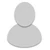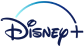## FANDOM

1,635 Pages

• So, that episode's on right now. How would you even go about quantifying the odds of rolling a number on the infinity-sided die? It's a fun little thought. I love math stuff. owo

• Well there's and infinite amount of numbers and an infinite amount of other things so it about 50/ 50 I guess

• The question is, is that really the case? I was discussing this with my cousin and one of my math professors and I came upon this logic. First of all, if you didn't know, there are two types of infinity: countable and uncountable. Sparing you the details, rational numbers are a countably infinite set and irrationals are uncountably infinite. And by their nature, there exist infinitely more irrational numbers than rational numbers. So if I were to pick a numbers at random of all the numbers in existence, the probability of pulling an irrational number is effectively 100%.

So with similar thinking, I figure it's fair to consider that you can assign a natural number to each of the infinite non-number events. If that's possible, then there is a countably infinite number of non-number events. This is versus the uncountably infinite set of all real numbers (not to mention imaginary and complex).

So by that, I argue that there is effectively a 100% chance of rolling a number rather than triggering an event. Granted, there's technically a chance of and non-number event happening, but it's an infinitely small chance, being effectively zero.

I like math. owo

• ....

When there is two different variables (here events and numbers) there cant be 100% of anything just saying

• That's where the term "effectively" comes into play. What I presented up there claims that there are infinitely more numbers than there are non-numbered events. If you take the limit of a/x as x approaches infinity, where a is some positive constant, you get zero. Technically, it never reaches 0, but it keeps getting closer and closer. So "at" infinity (you can never get to infinity as it's just a concept), you're looking at a number that's infinity small but still have value. (An infinitesimal is what this is called.) So there's an infinitely small chance that you can roll a non-number, but there's a 99.99999999999999999...% chance of rolling a number. (For the record, if you write 99.99999... as a rational number, it's 100.)

So I'm claiming the number of numbers dwarfs the number of non-numbers by this extreme. Basically, Ford got an extremely lucky roll in that episode such that he had an infinitely small chance within an infinitely small chance to bring those characters to life.

Of course, there are concepts in mathematics that I don't know like measure theory, which might apply here, so I could be off a tad.

• Also, just some quick math to clarify something I mentioned up there, let .9999...=x. Then 99.999....=100x. (Since the nines go off infinitely, you don't lose any decimal places.) So 100x-x=99 which means 99x=99 which means x=1. So .999999...=1 making 99.9999....=100.

• Derp I understand now :P

• Though now I'm talking with my math professor on Facebook and he's trying to explain to me why it would be a 0% probability instead. @.@

I love math, but sometimes theory stuff gets super confusing. xD;;

• Ikr. It's a theory because everyone gets too confused to solve it.

• Nah. I think this is something that can be quickly solved once you have the right theoretical groundwork. It just takes some higher level math. Probabilities with infinity are fun to consider. Like, if I randomly generated a number in the set of all real numbers, the odds of me picking any specific number is effectively 0% but the odds of me picking a number at all is a true 100%. The odds of me picking any kind of rational number is also effectively 0% so I'm infinitely more likely to pick an irrational number.

I'm assuming if I wanted to go into complex numbers, similar logic would lead me to believe I'd pick a complex number with both parts irrational. Though, as always, the specific number I pick would effectively have a 0% probability.

Also, still discussing this with my math professor. He misunderstood the concept of the question. He's the super nerdy type that pals around campus in Hawaiian shirts and will play people blind in chess while watching tv in the other room and win. So I trust he can help me understand this. xD;;

I discussed this with my math teacher today. She said to go back to the more basic second grade aspect of it. Like how they said in second grade 'if you have a bag full of jellybeans there is 20 of then 10 are grape 10 are orange. There is a 50/50 chance of pulling a grape one.' So that works as long as there is an equal number of each item... in this case there is. Infinite numbers and infinite events so therefore it's a 50/50 chance of rolling a number

• Except infinity of one thing isn't equal to infinity of another thing. Put simply, infinity is actually defined such that infinity minus 1 equals infinity. And as stated before, uncountably infinite is infinitely greater than countably infinite anyway.

You teacher should have had calculus in college. There's a reason why infinity divided by infinity doesn't equal one.

• I mean, the events that can occur are also infinite, so it could be infinitely small, since all the numbers in the world may be equivalent to "you do x", "y happens", "z occurs", etc.

• The chances of rolling a number would be 49.999...%. The error from op came from assuming there was a countably infinite amount of non-number events. This lead them to believe the chances of rolling an irrational number were infinitely greater, when they are, in actuality, virtually equal. For every irrational number that exists, there exist numbers like irrational numbers, roots of those numbers, reciprocals, etc, along with the probability that those number won't be rolled, but instead be spoken aloud when the die stops (technically a non-number event). Therefore, the amount of non-number events can be paired one-to-one with every number, with at least a countably infinite amount of possible events remaining.

• correction re: myself, there is a remaining uncountably infinite amount of possible events, rendering the chances of rolling a number 0%.

•Give Kudos to this message
You've given this message Kudos!
See who gave Kudos to this message
Community content is available under CC-BY-SA unless otherwise noted.